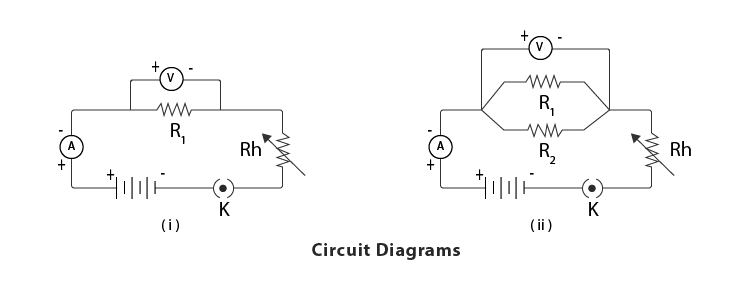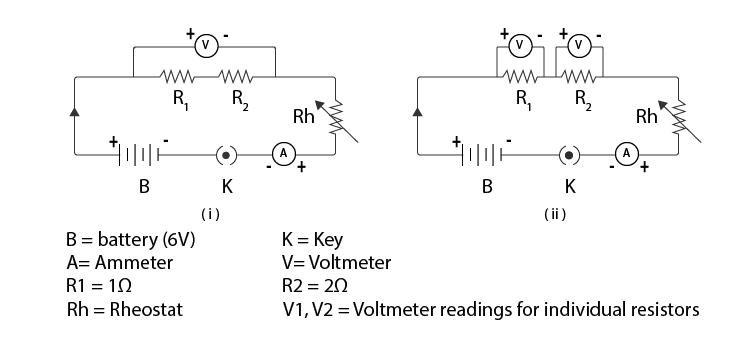Jet Set Go! All about Aeroplanes Jet Set Go! All about Aeroplanes

# Determination of the Equivalent Resistance of Two Resistors When Connected in Series and Parallel

Before we determine the value of the resistor in the circuit, it is necessary to understand how resistors are connected with each other. Resistors can be connected either in series or parallel combinations. In this article, let us know about the resistors in series and parallel combinations.

When resistors are connected end-to-end, they are said to be connected in a series combination. Consider two resistors, namely R1 and R2, connected in a series combination. The total voltage across the resistors is given by V = R1+R2.

When resistors are connected across each other in a circuit, they are said to be connected in parallel combination. Consider two resistors, namely R1 and R2, connected in parallel combinations. The total resistance across the voltage is given by 1/R = 1/R1+1/R2.

## a) Determination of the Equivalent Resistance of Two Resistors When Connected in Parallel

A circuit is said to be in a parallel connection when the resistors are connected in such a way that they branch out from one point. The potential difference in a parallel circuit is the same for each resistor and the current flow is not the same for all resistors. The total current flow through the circuit is calculated by summing up the current flow through each resistor. Parallel circuit connections find application in household electric distribution. The reason why a parallel circuit is preferred is to avoid short circuits and to monitor the flow of current for different devices. The other type of circuit is a series connection. Below is an experiment to determine the equivalent resistance when the resistors are connected in parallel.

### Aim

To determine the equivalent resistance of two resistors when connected in parallel.

### Theory

If the resistors are connected in parallel along with a battery, then the total current I is calculated as a sum of the separate value of current through each branch. It is given as:

I = I1+I2+I3+….

### Materials Required

1. A battery
2. A plug key
3. Connecting wires
4. An ammeter
5. A voltmeter
6. Rheostat
7. A piece of sandpaper
8. Two resistors of different values### Procedure

1. Make all the connections as shown in the experimental setup I by keeping the key off.
2. Insert the key when the circuit is connected appropriately.
3. For resistors R1 and R2, note three readings of ammeter and voltmeter.
4. Connect the circuit as shown in the experimental setup II.
5. Resistors and voltmeter both are connected in parallel.
6. Record three readings of ammeter and voltmeter and use a rheostat.
7. Remove the key.
8. With the help of the observation table, do the calculations.

### Observation Table

 Resistor used No.of observations Voltmeter reading in Volts (V) Ammeter reading in Ampere (I) R=V/I (in Ohm) Mean value of resistance (Ohm) R1 (first resistor) a 0.01 0.01 1 R1 = 1 ohm b 0.02 0.02 1 c 0.04 0.04 1 R2 (second resistor) a 0.02 0.01 2 R2 = 2 ohm b 0.06 0.03 2 c 0.08 0.04 2 1/Rp=(1/R1)+(1/R2) Parallel combination a 0.026 0.04 0.67 Rp=0.67 ohm 1/Rp=1.5 ohm

### Result

 The calculated value of $$\begin{array}{l}\frac{1}{R_{p}}\end{array}$$ $$\begin{array}{l}\frac{1}{R_{p}}=(\frac{1}{R_{1}})+(\frac{1}{R_{2}})=1.5\Omega\end{array}$$ The experimental value of $$\begin{array}{l}\frac{1}{R_{p}}\end{array}$$ $$\begin{array}{l}\frac{1}{R_{p}}=1.5\Omega\end{array}$$ The equivalent resistance Rp is less than the individual resistance.

### Precautions

1. The connecting wires used should be thick copper wire and using sandpaper, the insulation at the end of the wires should be removed.
2. The connections should be tight to avoid introducing external resistance.
3. To make connections, the circuit diagram should be referred to.
4. To make the current entry from the positive terminal and exit from the negative terminal, the ammeter should be connected in series.
5. Resistor and voltmeter should be connected in parallel.
6. The least count of ammeter and voltmeter should be calculated properly.
7. When there is no current flow, the ammeter and voltmeter should be at zero.
8. To avoid heating and change the resistor’s resistance value, the current should flow while taking the readings.

## Viva Questions

Q1. What happens to the total resistance when resistors are combined in parallel?

Ans: When the resistors are combined in parallel, the total resistance will decrease.

Q2. When the resistors are connected in parallel, name the constant physical quantity.

Ans: The physical quantity that remains constant when the resistors are connected in parallel is the voltage.

Q3. How to calculate equivalent resistance when three resistors are connected in parallel?

Ans: Following is the formula used to calculate the equivalent resistance when three resistors are connected in parallel:

 $$\begin{array}{l}\frac{1}{R_{p}}=\frac{1}{R_{1}}+\frac{1}{R_{2}}+\frac{1}{R_{3}}\end{array}$$

Q4. What will be the net resistance if two resistors with 3 Ω and 6 Ω resistance are connected in parallel?

Ans: The net resistance of the resistors connected in parallel is given as:

$$\begin{array}{l}\frac{1}{R_{p}}=\frac{1}{R_{1}}+\frac{1}{R_{2}}\end{array}$$
$$\begin{array}{l}\frac{1}{R_{p}}=\frac{1}{3}+\frac{1}{6}\end{array}$$
$$\begin{array}{l}=\frac{3}{6}\end{array}$$
$$\begin{array}{l}\Rightarrow R_{p}=\frac{6}{3}=2\Omega\end{array}$$

Q5. What would be the total resistance when the resistors are connected in parallel with resistors value 3Ω?

Ans: The total resistance of the resistors connected in parallel is given as:

$$\begin{array}{l}\frac{1}{R_{p}}=\frac{1}{R_{1}}+\frac{1}{R_{2}}+\frac{1}{R_{3}}\end{array}$$
$$\begin{array}{l}\frac{1}{R_{p}}=\frac{1}{3}+\frac{1}{3}+\frac{1}{3}=\frac{3}{3}=1\end{array}$$
$$\begin{array}{l}\Rightarrow R_{p}=1\Omega\end{array}$$

## b) Determination of the Equivalent Resistance of Two Resistors When Connected in Series

In a series connection, the current flows through one resistor and then the other. The current flowing through each resistor is the same as the current flows in one direction. The total voltage is the sum of all the voltage drops across the resistors. There are few drawbacks of a series circuit, and they are:

• Either because of the overflow of current or loss of current, short-circuiting may happen.
• The electrical appliance may be damaged due to a high current flow.
• Only one device cannot be switched off without turning off both devices.

### Aim

To determine the equivalent resistance of two resistors when connected in series.

### Theory

The resistance can be increased or decreased depending on the combination and connections in a circuit. The difference between the series and parallel circuit is based on the arrangement of the resistors. Resistors are said to be connected in series if their ends are joined. The potential difference across each resistor would be different, but the current would be the same.

If two resistors are connected in series, then;

Resistance, R = R1+R2

Current, I = constant

Potential difference, V = V1+V2

On applying Ohm’s law, we get,

V1 = IR1

V2 +IR2

V = V1+V2

V = I(R1+R2)

∴ R = R1+R2

### Materials Required

• Two resistors of different values
• A battery of 6 volts
• Ammeter
• Plug key
• Connecting wires
• A piece of sandpaper
• Voltmeter
• Rheostat

### Circuit Diagram### Procedure

1. With the help of a circuit diagram, make the connections.
2. Do not switch on the key.
3. The ammeter should be connected in series, the voltmeter in parallel, and the rheostat in series.
4. Make the connections as shown in the experimental setup and check of +ve and -ve terminals of the battery.
5. By inserting the key, record the ammeter and voltmeter readings.
7. Note down the readings of a voltmeter by connecting it to each resistor.
8. Measure the potential difference, V1 across the first resistor by plugging in the key.
9. Measure the potential difference, V2 across the second resistor by plugging in the key.
10. Calculate the relationship between V, V1, and V2.

### Observation Table

 Resistor used No.of observations Voltmeter reading in Volts (V) Ammeter reading in Ampere (I) R = V/I (in ohm) Mean value of resistance (ohm) R1 (first resistor) a 0.01 0.01 1 $$\begin{array}{l}R_{1}=\frac{1+1+1}{3}=\frac{3}{3}=1\;ohm\end{array}$$ b 0.02 0.02 1 c 0.04 0.04 1 R2 (second resistor) a 0.02 0.01 2 $$\begin{array}{l}R_{1}=\frac{2+2+2}{3}=\frac{6}{3}=2\;ohm\end{array}$$ b 0.06 0.03 2 c 0.08 0.04 2 Rs=R1+R2 (series combination) a 0.03 0.01 3 $$\begin{array}{l}R_{1}=\frac{3+3+3}{3}=\frac{9}{3}=3\;ohm\end{array}$$ b 0.06 0.02 3 c 0.09 0.03 3

### Result

 The calculated value of Rs Rs=R1+R2=3Ω The experimental value of Rs 3Ω

Hence, it is verified that Rs=R1+R2.

### Precautions

1. Voltmeter and resistor should always be in parallel.
2. The least count of voltmeter and ammeter should be calculated properly.
3. Connections should be as per the experimental setup.
4. When no current flows through the ammeter and voltmeter, the pointers should be at zero.
5. The connecting wires that are used should be thick copper wire and using sandpaper the insulation at the end of the wires should be removed.
6. The connections should be tight to avoid introducing external resistance.
7. To avoid heating and change the resistor’s resistance value, the current should flow while taking the readings.

## Viva Questions

Q1. What will be the total resistance of two resistors connected in series with values 2 Ω and 3 Ω?

Ans: The total resistance is given as:

R = R1 + R2

∴ R = 2 Ω + 3 Ω = 5 Ω

Q2. Determine the voltmeter reading for a series circuit if a current of 5A is flowing through 2 Ω and 3 Ω resistors.

Ans: The voltmeter reading across the 2 Ω resistor is given as:

V =IR

V1= 5 × 2 = 10V

The voltmeter reading across the 3 Ω resistor is given as:

V =IR

V2= 5 × 3 = 15V

∴ V = V1+V2

V = 10 + 15 = 25V

Q3. How should the resistor be connected so that the resultant resistance is increased?

Ans: To get the resultant resistance maximum, the resistors should be connected in series.

Q4. What will total resistance be if two equal resistors are connected in series?

Ans: If two equal resistors are connected in series, then the total resistance is given as:

R + R = 2R.

Q5. Where should the voltmeter be connected when the resistors are in series?

Ans: The voltmeter should be connected parallel when the resistors are in series.

## Watch the video and learn to find the equivalent resistance in different circuit arrangements